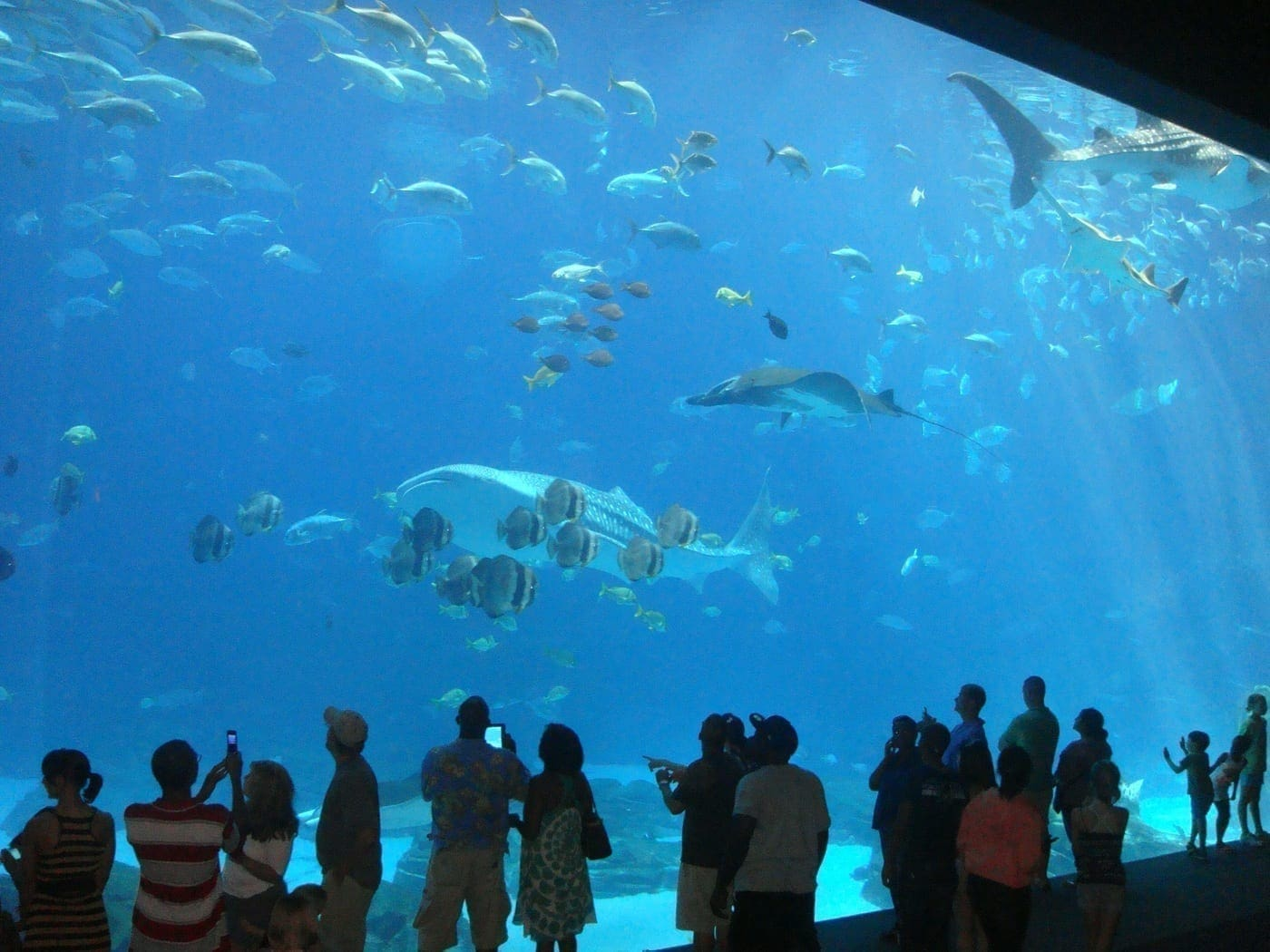# How to Learn about Creation in the Real World[Originally published as Math at the Aquarium]

My husband and I recently visited the Georgia Aquarium, and I was struck by how much math is used in caring for and describing the characteristics of sea creatures. Below are a couple of examples. I’ve tried to word them as actual problems for different ages. I hope you enjoy working through them with your students! (The answers are below.)

• How Big Is That Tank? (All ages)
• Elementary Problem: Let’s say there’s a tank that is approximately the size of a football field (which the biggest one at the Georgia Aquarium is nearly such a size). Football fields are about 100 yd long by about 50 yd. Let’s say this tank is 30 ft, or 10 yd, deep. Using these estimates of the size of the tank, find the approximate volume in cubic yards if it is a gigantic rectangular prism. (The volume is found by multiplying the length times the width times the depth.)
• Junior High Problem: Given the information in the elementary problem, about how many gallons does the tank hold? 1 yd equals 36 in, and 231 cubic inches equals 1 gallon.
• Algebra Problem: We found out online that a tank at the Georgia Aquarium holds about 6,300,000 gallons of water. Given that the volume of a rectangular prism is V = Bh, where B is the area of the rectangular base and h is the height, and that a tank is a rectangular prism, use algebra to find the area of the rectangular base (B) of the tank if the depth of the tank is 10 yd. Give your answer in square feet. What percentage of the area of a football field is this if the area of a football field is 45,000 ft2Hint: You will need to first convert all the measurements to cubic inches. Then solve for B. 1 yd equals 36 in, 1 ft equals 12 in, and 231 cubic inches equals 1 gallon.
• How Much Do Those Sea Lions Eat? (Upper Elementary and Above) – In the show, we were told that each sea lion eats a certain percentage of its body weight each day that is close to 5%.
• If a sea lion weighs 500 lb, how many lb of food does it need each day?
• What about if it grows to 800 lb?
• If you were in charge of ordering the sea lion fish to eat, how many pounds would you need to order per day if you needed food for a 400 lb and a 750 lb sea lion?

Now, there’s a lot more math involved at the aquarium. (Measuring the temperature of the tanks to make sure it is what the animals need, figuring out how many fish can be in one tank, etc.) But hopefully you enjoyed this little glimpse into aquarium math.

Remember, math is much more than a textbook exercise—it is a real-life “tool”…and one that works because of God’s faithfulness.

How Big Is That Tank?

• Elementary Problem: (100 yd)(50 yd)(10 yd) = 50,000 yd3
• Junior High Problem: Converting cubic yards to cubic feet: 50,000 yd3(36 in/1 yd)(36 in/1 yd)(36 in/1 yd) = 2,332,800,000 in3
Converting cubic inches to gallons: 2,332,800,000 in3(1 gal/231 in3) = 10,098,701.3 gal
Note: The 50,000 ydcame from the answer to the elementary problem.
• Algebra Problem: Converting the volume to cubic inches: 6,300,000 gal(231 in3/1 gal)=1.4553 x 10in3
Converting the depth to inches: 10 yd(36 in/1 yd) = 360 in
Solving the formula for BV = Bh
Diving both sides by -> B=V/h
Plug in the values: B = 1.4553 x 10 in3/360 in = 4.0425 x 10 in2
Convert the final answer to square feet: = 4.0425 x 10 in2(1 ft/12 in)(1 ft/12 in) = 28,072.91667 ft2
The percentage of the area of a foot field is P = 100 % (28,072.91667 ft2/45,000 ft2) = 62.38 %

How Much Do Those Sea Lions Eat?

• 0.05(500 lb) = 25 lb
• 0.05(800 lb) = 40 lb
• 0.05(400 lb) + 0.05(750 lb) = 20 lb + 37.50 lb = 57.50 lb per day

[Editor’s note: If it takes math to provide enough water and food for the creatures already there, how much mathematical (physics, chemistry, etc.) planning did it take to move these whale sharks?]

## Written by Kate [Loop] Hannon

After having her own view of math transformed, Katherine has been researching, writing, and speaking on a biblical worldview of math for more than a decade. She's the author of the popular Principles math curriculum series published by Master Books. Her books on math and a biblical worldview have been used by individuals, homeschool groups, and Christian schools and colleges. Receive a free video on transforming math at www.christianperspective.net/math/transforming-math

1. Wow, there is a lot of math behind designing, building and maintaining an aquarium! But yet we’re supposed to believe that our world’s oceans, tides, rivers, lakes and complex watersheds all happened by random chance???? It just “doesn’t add up”! 1 Corinthians 1:19 “For it is written, I will destroy the wisdom of the wise,”